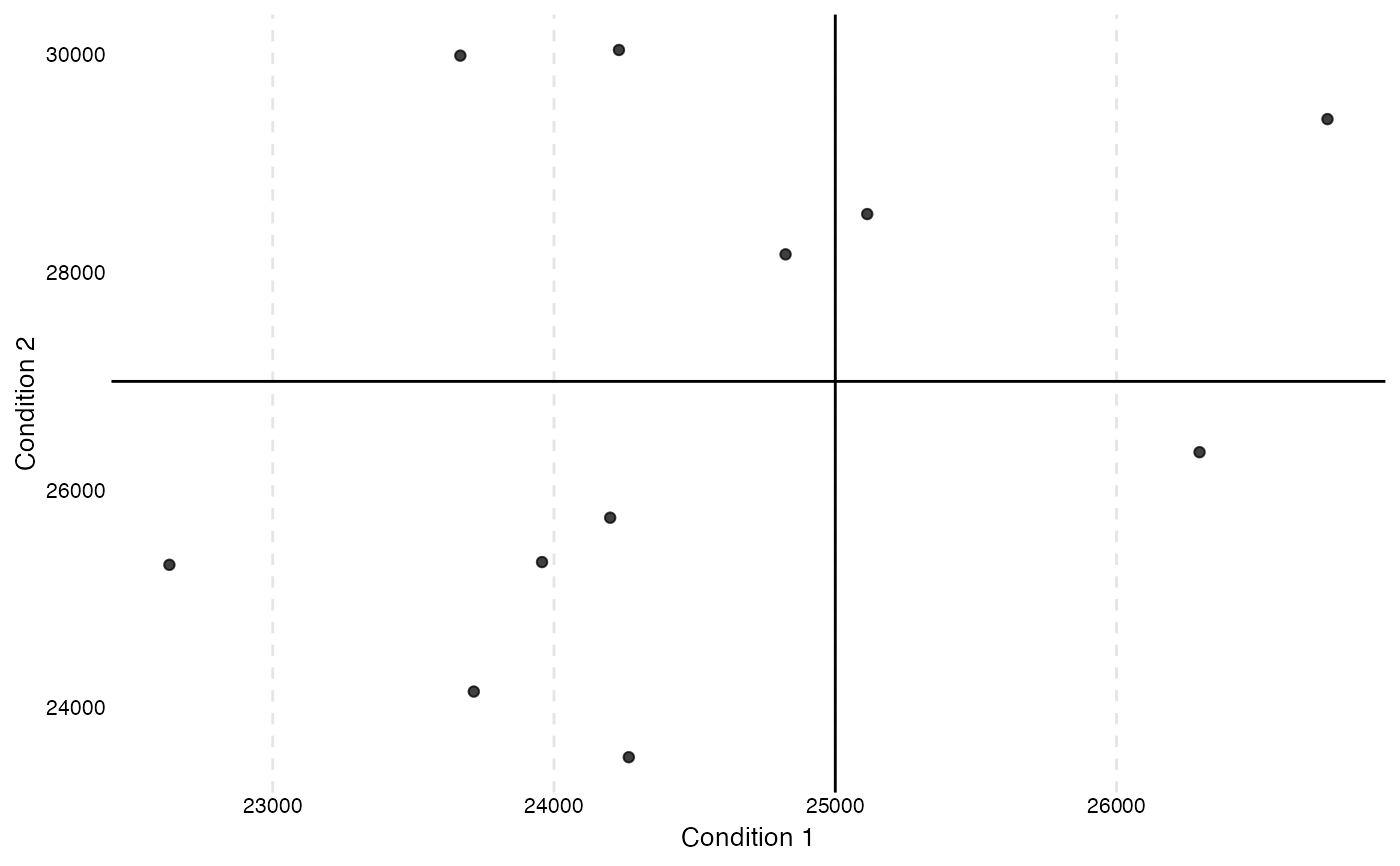This function plots a ggplot to visualize a cross table plot.

## Usage

overview_crossplot(
dat,
id,
time,
cond1,
cond2,
threshold1,
threshold2,
xaxis = "Condition 1",
yaxis = "Condition 2",
label = FALSE,
color = FALSE,
dot_size = 2,
fontsize = 2.5
)

## Arguments

dat

id

Your scope (e.g., country codes or individual IDs). If the id variable contains NAs, they will not be included in the plot.

time

Your time (e.g., time periods given by years, months, ...)

cond1

Variable that describes the first condition

cond2

Variable that describes the second condition

threshold1

A threshold for cond1

threshold2

A threshold for cond2

xaxis

Label of the x axis ("Condition 1" is default)

yaxis

Label of the y axis ("Condition 2" is default)

label

Label of the observations. Overlapping labels are avoided by using 'ggrepel'

color

Color of the different observation groups

dot_size

Option argument that defines the dot size (default is 2)

fontsize

If label is TRUE, the fontsize arguments allows to define the text of the labels (the default is 2.5)

## Value

A ggplot figure that presents the sample information visually in a cross table

## Examples

data(toydata)
overview_crossplot(
dat = toydata,
cond1 = gdp,
cond2 = population,
threshold1 = 25000,
threshold2 = 27000,
id = ccode,
time = year
)